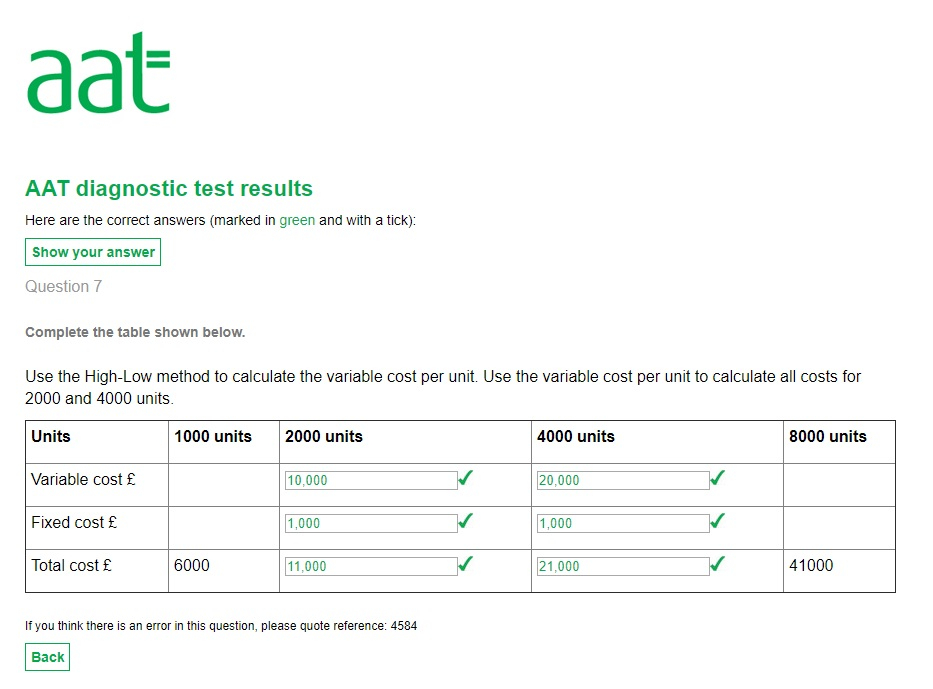# High Low

Hi there,

I would just like to know how I find the answers listed in this screenshot. I spent about 20 minutes doing this and got nowhere.

Thanks,

Gareth• We need to find the variable and the fixed element
41000-6000=£35000
8000-1000=7000units
£35000/7000units=£5 cost of variable element

1000units x 5=£5000 variable element
Total cost less variable cost equals fixed cost
6000-5000=1000 fixed cost

Check

8000units x£5=£40000 + £1000= £41000

2000units x 5 =10000 variable
10000 +1000 fixed = 11000 toatal cost

4000x5=20000 + 1000 fixed=21000
AAT Level 2. 2018 Distinction
AAT Level 3. 2018 Distinction
AAT Level 4. 2019 FSLC 100% MABU 93% MADC 82% PDSY 80%
• Awesome, thanks a lot. That all makes sense now